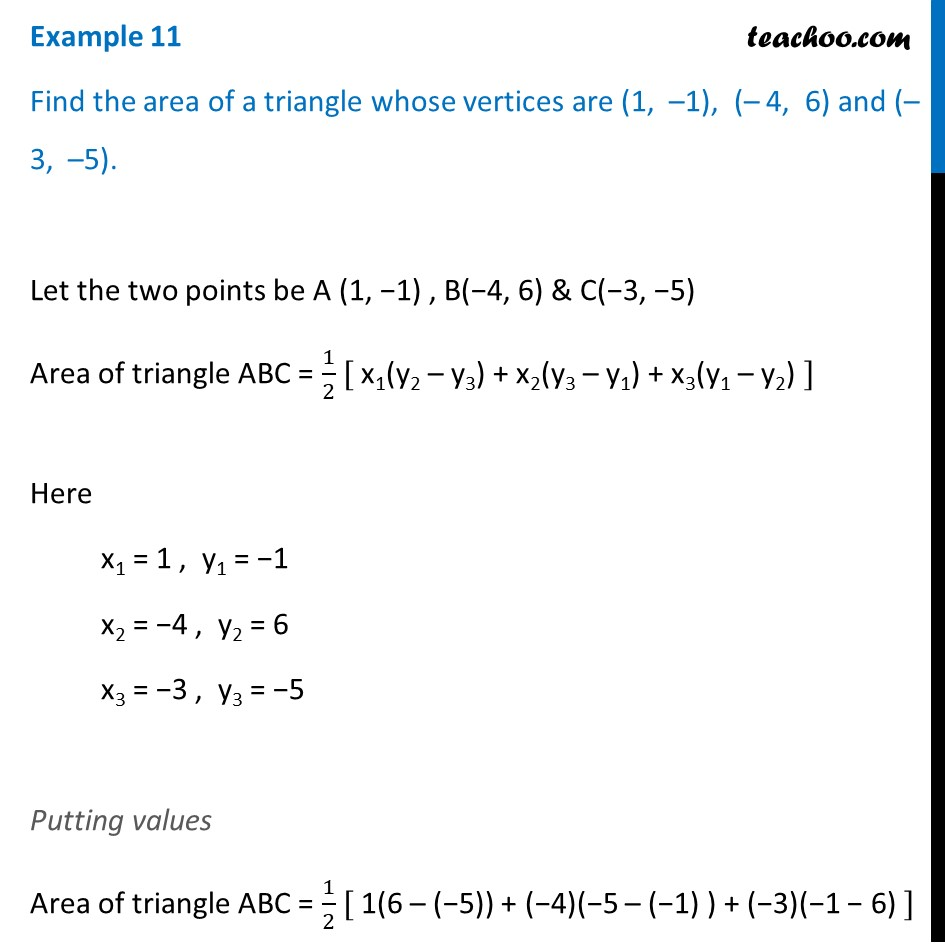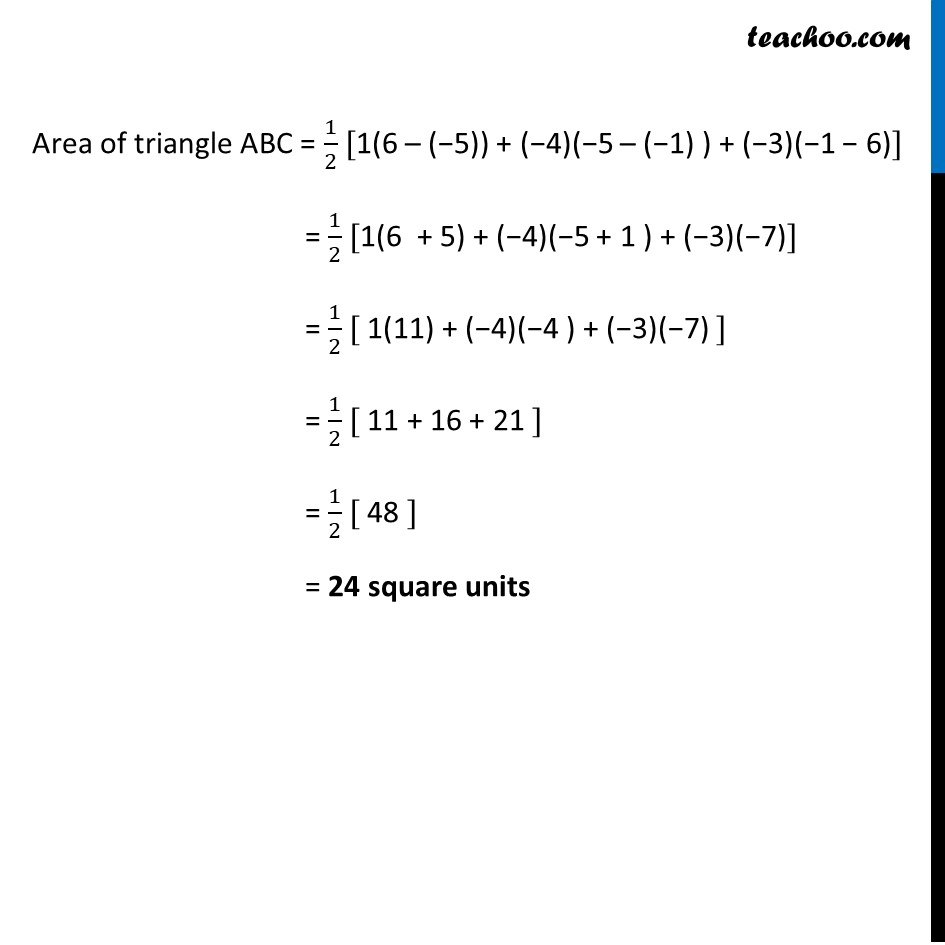Area of triangle

Chapter 7 Class 10 Coordinate Geometry
Concept wiseLearn in your speed, with individual attention - Teachoo Maths 1-on-1 Class

### Transcript

Question 1 Find the area of a triangle whose vertices are (1, –1), (– 4, 6) and (–3, –5). Let the two points be A (1, −1) , B(−4, 6) & C(−3, −5) Area of triangle ABC = 1/2 [ x1(y2 – y3) + x2(y3 – y1) + x3(y1 – y2) ] Here x1 = 1 , y1 = −1 x2 = −4 , y2 = 6 x3 = −3 , y3 = −5 Putting values Area of triangle ABC = 1/2 [ 1(6 – (−5)) + (−4)(−5 – (−1) ) + (−3)(−1 − 6) ] Area of triangle ABC = 1/2 [1(6 – (−5)) + (−4)(−5 – (−1) ) + (−3)(−1 − 6)] = 1/2 [1(6 + 5) + (−4)(−5 + 1 ) + (−3)(−7)] = 1/2 [ 1(11) + (−4)(−4 ) + (−3)(−7) ] = 1/2 [ 11 + 16 + 21 ] = 1/2 [ 48 ] = 24 square units